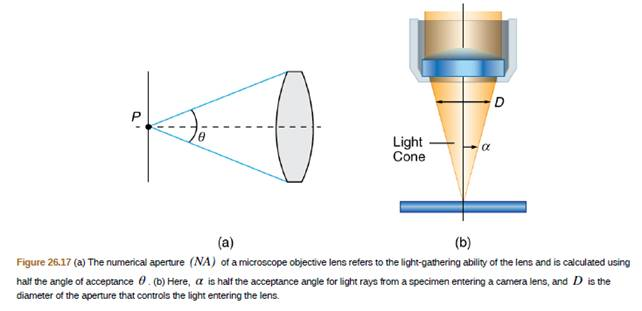Chapter 26, Problem 31PE

Chapter
Section
Textbook Problem

You are using a standard microscope with a 0.10 N A     4 X objective and switch to a 0.65 N A     40 X objective. What are the acceptance angles for each? Compare and comment on the values. Which would you use first to locate the target area on of your specimen? (See Figure 26.17.)To determine

What are the acceptance angles for each? Compare and comment on the values. Which would you use first to locate the target area on of your specimen?

Explanation

Given info:

n=1.00NA1=0.10NA2=0.65

Formula used:

The acceptance angle θ can be obtained using:

NA=nsinθ2

Isolatinggets:

θ=2sin1NAn

Calculation:

The first acceptance anglegives:

&ems

Still sussing out bartleby?

Check out a sample textbook solution.

See a sample solution

The Solution to Your Study Problems

Bartleby provides explanations to thousands of textbook problems written by our experts, many with advanced degrees!

Get Started